Updating search results...

# 42 Results

View
Selected filters:
• NC.M1.A-REI.3Rating
0.0 stars

Sample Item provided by Smarter Balanced as preliminary examples of the types of items that students might encounter on the summative assessment. The item assesses student understanding of solving linear inequalities in one-variable. MAT.HS.TE.1.0AREI.I.088

Subject:
Math 1
Mathematics
Material Type:
Activity/Lab
Assessment
Provider:
Oregon Department of Education
Author:
Smarter Balanced Assessment Consortium
02/26/2019Conditional Remix & Share Permitted
CC BY-NC-SA
Rating
0.0 stars

A work in progress, CK-12's Algebra I Second Edition is a clear presentation of algebra for the high school student. Topics include: Equations and Functions, Real Numbers, Equations of Lines, Solving Systems of Equations and Quadratic Equations.

Subject:
Mathematics
Material Type:
Textbook
Provider:
CK-12 Foundation
Provider Set:
CK-12 FlexBook
Author:
Andrew
Annamaria
Anne
Eve
Farbizio
Gloag
Rawley
12/03/2010Conditional Remix & Share Permitted
CC BY-NC-SA
Rating
0.0 stars

CK-12 Foundation's Basic Algebra FlexBook is an introduction to the algebraic topics of functions, equations, and graphs for middle-school and high-school students.

Subject:
Mathematics
Material Type:
Textbook
Provider:
CK-12 Foundation
Provider Set:
CK-12 FlexBook
Author:
Farbizio, Annamaria
Gloag, Andrew
Gloag, Anne
Kramer, Melissa
09/21/2010Conditional Remix & Share Permitted
CC BY-NC-SA
Rating
0.0 stars

Students understand that an equation is a statement of equality between two expressions. When values are substituted for the variables in an equation, the equation is either true or false. Students find values to assign to the variables in equations that make the equations true statements.

Subject:
Math 1
Mathematics
Material Type:
Lesson
Author:
EngageNY
02/02/2020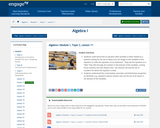Conditional Remix & Share Permitted
CC BY-NC-SA
Rating
0.0 stars

Students understand that an equation with variables is often viewed as a question asking for the set of values one can assign to the variables of the equation to make the equation a true statement. They see the equation as a “filter” that sifts through all numbers in the domain of the variables, sorting those numbers into two disjoint sets: the Solution Set and the set of numbers for which the equation is false.

Students understand the commutative, associate, and distributive properties as identities, e.g., equations whose solution sets are the set of all values in the domain of the variables.

Subject:
Math 1
Mathematics
Material Type:
Lesson
Author:
EngageNY
02/02/2020Conditional Remix & Share Permitted
CC BY-NC-SA
Rating
0.0 stars

Students learn if-then moves using the addition and multiplication properties of inequality to solve inequalities and graph the solution sets on the number line.

Subject:
Math 1
Mathematics
Material Type:
Lesson
Author:
EngageNY
02/02/2020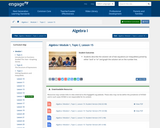Conditional Remix & Share Permitted
CC BY-NC-SA
Rating
0.0 stars

Students describe the solution set of two equations (or inequalities) joined by either “and” or “or” and graph the solution set on the number line.

Subject:
Math 1
Mathematics
Material Type:
Lesson
Author:
EngageNY
02/02/2020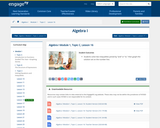Conditional Remix & Share Permitted
CC BY-NC-SA
Rating
0.0 stars

Students solve two inequalities joined by “and” or “or,” then graph the solution set on the number line.

Subject:
Math 1
Mathematics
Material Type:
Lesson
Author:
EngageNY
02/02/2020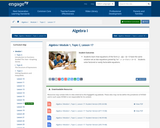Conditional Remix & Share Permitted
CC BY-NC-SA
Rating
0.0 stars

Students learn that equations of the form (x - a)(x - b) = 0 have the same solution set as two equations joined by “or:” x - a = 0 or x - b = 0. Students solve factored or easily factorable equations.

Subject:
Math 1
Mathematics
Material Type:
Lesson
Author:
EngageNY
02/02/2020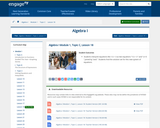Conditional Remix & Share Permitted
CC BY-NC-SA
Rating
0.0 stars

Students interpret equations like 1/x = 3 as two equations “1/x = 3 ” and “x ≠ 0 ” joined by “and.” Students find the solution set for this new system of equations.

Subject:
Math 1
Mathematics
Material Type:
Lesson
Author:
EngageNY
02/02/2020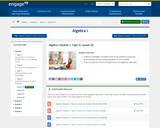Conditional Remix & Share Permitted
CC BY-NC-SA
Rating
0.0 stars

Students investigate a problem that can be solved by reasoning quantitatively and by creating equations in one variable.
They compare the numerical approach to the algebraic approach.

Subject:
Math 1
Mathematics
Material Type:
Lesson
Author:
EngageNY
02/02/2020Rating
0.0 stars

Using tiles to represent variables and constants, learn how to represent and solve algebra problem. Solve equations, substitute in variable expressions, and expand and factor. Flip tiles, remove zero pairs, copy and arrange, and make your way toward a better understanding of algebra.

Subject:
Math 1
Math 2
Math 3
Mathematics
Material Type:
Interactive
Provider:
National Council of Teachers of Mathematics
Author:
Illuminations
02/26/2019Rating
0.0 stars

This lesson unit is intended to help you assess how well students are able to create and solve linear and non-linear equations. In particular, the lesson will help identify and help students who have the following difficulties: â€¢ Solving equations where the unknown appears once or more than once. â€¢ Solving equations in more than one way

Subject:
Mathematics
Material Type:
Lesson Plan
Provider:
Mathematics Assessment Project
11/09/2017Conditional Remix & Share Permitted
CC BY-NC-SA
Rating
0.0 stars

CK-12 Foundation's Algebra FlexBook is an introduction to algebraic concepts for the high school student. Topics include: Equations & Functions, Real Numbers, Equations of Lines, Solving Systems of Equations & Quadratic Equations.

Subject:
Mathematics
Material Type:
Textbook
Provider:
CK-12 Foundation
Provider Set:
CK-12 FlexBook
12/06/2018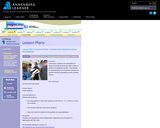Rating
0.0 stars

Students use manipulatives to represent visually the steps they take to obtain a solution to an algebraic equation. They develop an understanding of the connections between the solution involving manipulatives and the symbolic solution.

Subject:
Math 1
Mathematics
Material Type:
Activity/Lab
Lesson Plan
Provider:
Annenberg Learner
Author:
Annenberg Learner
02/26/2019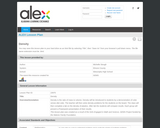Rating
0.0 stars

In this lesson, density will be introduced to students by a demonstration of coke verses diet coke. The teacher will then solve density problems for the students on the board. The class will then complete a lab on the density of plastics. After lab the students will compare results. Each group presents a PowerPoint presentation of their results.

Subject:
Math 1
Mathematics
Material Type:
Activity/Lab
Lesson Plan
Provider:
Alabama Dept.of Education
Author:
Michelle Stough
02/26/2019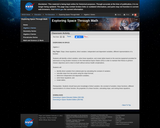Unrestricted Use
Public Domain
Rating
0.0 stars

EXERCISING IN SPACE

Algebra 1

Key Topic: Slope, linear equations, direct variation, independent and dependent variables, different representations of a function

Students will identify a direct variation, solve linear equations, and create tables based on the exercise equipment provided for astronauts on long duration missions on the International Space Station (ISS) in order to maintain their fitness to perform mission objectives and to return to Earth without serious health complications.

Students will
identify direct variation from ordered pairs by calculating the constant of variation;
calculate slope from two points using the slope formula;
determine independent and dependent variables;
solve linear equations; and
create tables.

Prerequisites: Students should have prior knowledge of direct variation, the constant of variation, linear functions, different representations of a linear function, the properties of a linear function, calculating slope, and solving linear equations.

Subject:
Algebra
Mathematics
Material Type:
Lesson
Author:
NASA
07/22/2020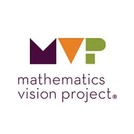Conditional Remix & Share Permitted
CC BY-NC-SA
Rating
0.0 stars

The purpose of this task is to apply the equation solving process developed in the previous task to solving literal equations and formulas. Working with literal equations solidifies the notion that operations have to be “un-done” in an appropriate order by doing the inverse operation to both sides of the equation. This task also solidifies the meaning of expressions by attending to the units associated with each of the variables.

Subject:
Math 1
Mathematics
Material Type:
Lesson
Author:
Mathematics Vision Project
03/10/2020Unrestricted Use
CC BY
Rating
0.0 stars

The purpose of this task is to solidify student understanding of geometric sequences to
find missing terms in the sequence. Students will draw upon their previous work in using tables
and writing explicit formulas for geometric sequences.

Subject:
Math 1
Mathematics
Material Type:
Activity/Lab
Author:
The Mathematics Vision Project
02/23/2020Conditional Remix & Share Permitted
CC BY-NC-SA
Rating
0.0 stars

The purpose of this task is to challenge students to reason about inequality relationships and to develop an understanding of the properties of inequalities. Each problem requires reasoning about numbers, including negative numbers and fractions, and thinking mathematically about the various possibilities in the given problem situation.

Subject:
Math 1
Mathematics
Material Type:
Lesson
Author:
Mathematics Vision Project